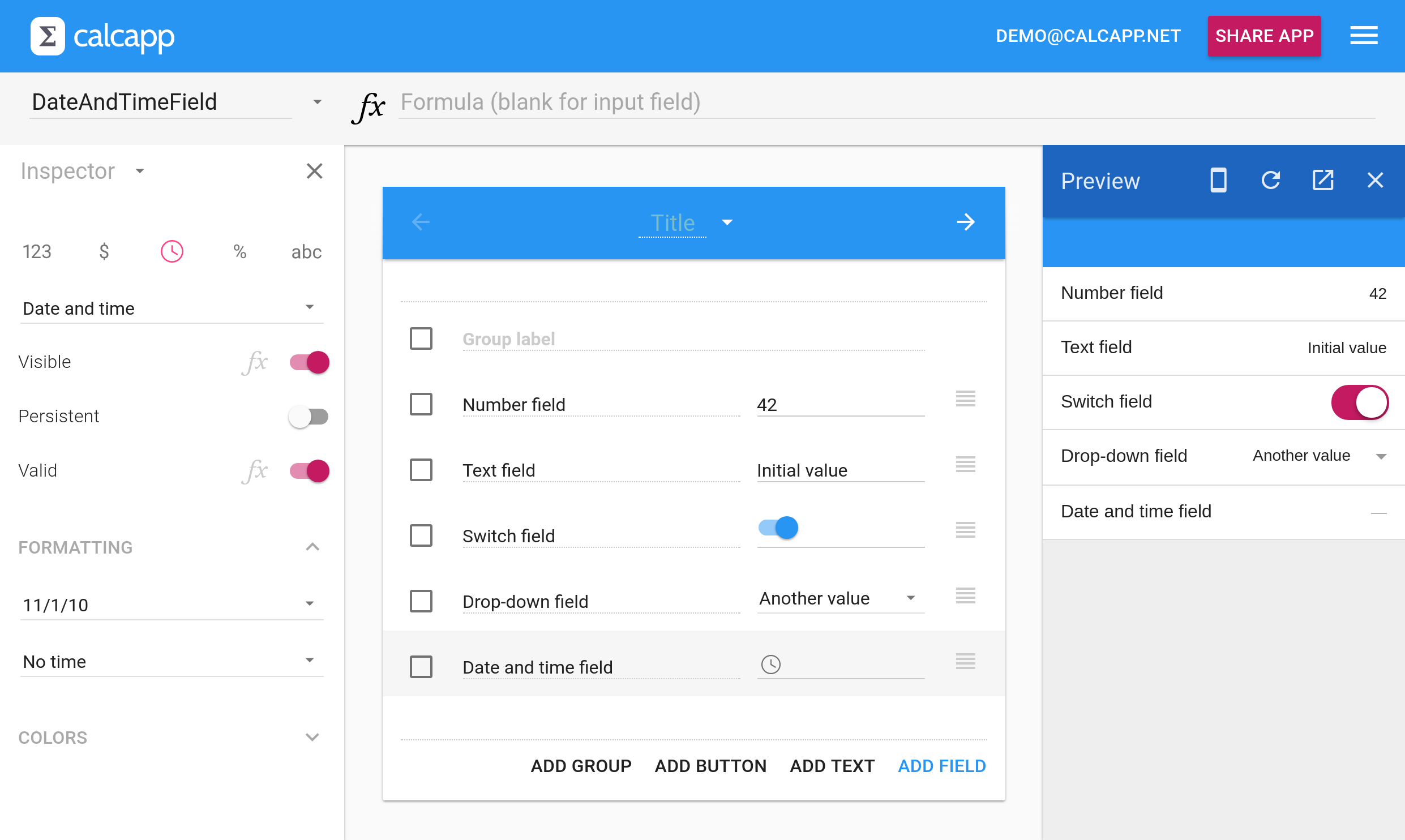• Learn
• Blog
• Pricing

# Tip: Assign default values to date and time fields

Date and time fields can be instructed to display a default value that appears when a user opens your app. You need to use formulas to set these values. This tip contains a number of useful such formulas, including one that selects a date seven days after the current date as the initial value.

Most fields can be instructed to display a certain value when the user opens your app. You can determine the number a number field is initially displayed with, the text string a text field shows initially and whether a switch field is toggled on or off. It also works for drop-down fields — instead of displaying an empty selection, your drop-down fields can display one of its values initially.

You select an initial value for a field simply by typing the value into the field itself in Calcapp Creator. (Bonus tip: You write “42” to have that number be the initial value for a number field, but you can also write something like “42 pounds” to include a unit.)

Here are a number of fields which have been initialized using this mechanism:All fields above show a default, initial value except for the date and time field. Date and time fields can’t be easily initialized to an unchanging date and time because we felt that it wouldn’t be useful. If you want to provide a default value for a date and time field, wouldn’t it be more useful to choose a date that is relative to the current date? You could then select a date seven days into the future or January 1 of the current year, for instance.

To do so, you associate a formula with the InitialValue property of the date and time field. Read this tip to learn more about associating a formula with the InitialValue property.

## Selecting dates using formulas

Here’s the formula you should associate with the InitialValue property of a date and time field to select a date seven days after the current date:

TODAY() + 7

The `TODAY` formula function returns today’s date. Due to the way dates work, adding seven to today’s date returns a date seven days after the current date. (Be sure to check out all the useful date and time functions in the reference sidebar.)

What about selecting January 1 of the current year? Simple:

DATE(YEAR(TODAY()), 1, 1)

The `DATE` formula function accepts three parameters: the year, month and day, in that order. The `YEAR` function returns the year from a date. When invoked with the current date, it extracts the year. As a result, the formula above returns a date corresponding to January 1 of the current year.

DATE(YEAR(TODAY()) - 1, MONTH(TODAY()), DAY(TODAY()))

The `MONTH` and `DAY` functions are equivalent to the `YEAR` function, but return numbers corresponding to the month and day of a date, respectively.

Finally, if you really want a date and time field to display an unchanging, constant date, just use the DATE function with parameters corresponding to the desired day:

DATE(2017, 10, 25)

## Selecting times using formulas

If you need to manipulate times instead of dates, the relevant functions are `NOW` (equivalent to `TODAY`), `TIME` (equivalent to `DATE`), `HOUR`, `MINUTE` and `SECOND` (equivalent to `YEAR`, `MONTH` and `DAY`.)

To display today’s date at 6pm, use this formula:

TODAY() + TIME(18, 0, 0)

The parameters of the `TIME` function are the hour, minute and second, where the hour is given using a 24-hour clock (also known as military time).

To display the time two hours earlier, use this formula:

NOW() - TIME(2, 0, 0)

Unlike the `TODAY` function, the `NOW` function also returns the time. To select a time two hours earlier, we subtract the return value of `TIME(2, 0, 0)` from the value returned from the `NOW` function.

Do you want to share a tip with other Calcapp users through this blog? Let us know!